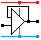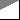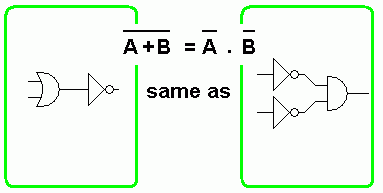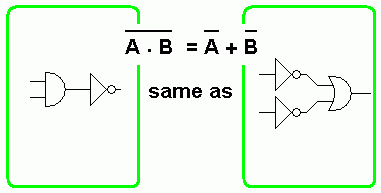Home

rOm
Quest
Glossary

Random
Page
Search
Site
Lush
Sim
Class
Subject
Images

Help
FAQ
Sign
Up
Log
In
 AS Level     Logic     >Algebra<     Bistable Latch     Karnaugh Maps     Multiplexing     NOR Array     Propagation Delay

# Logic Algebra

Site for Eduqas/WJEC - Go to the AQA site.

AS Level    Combinational LogicQuestions 0 to 26   -->  View All

## Techniques

If you have a good understanding of the logic gate rules, it is possible to work out a simpler solution just by examining the truth table and thinking hard. But this is a rather hit and miss approach and Karnaugh maps and Boolean algebra can be faster and more reliable methods but neither is easy.

Boolean algebra can be used to simplify expressions/circuits to generate a more optimal solution.

## De Morgan Boolean Identities

• A + B = A . B• A . B = A + B## De Morgan

• A + B = A . B
• A . B = A + B

## Gate Behaviour

• AND GATE
• A . 1 = A        The gate is open.
• A . 0 = 0        The gate is closed - output low.
• OR GATE
• A + 1 = 1        The gate is closed - output high.
• A + 0 = A        The gate is open.

## Commutative

• When using AND and OR, it makes no difference if the inputs are swapped.
• A . B = B . A
• A + B = B + A

## Associative

• The presence or absence of brackets makes no difference.
• A . (B . C) = (A . B) . C = A . B . C
• A + (B + C) = (A + B) + C = A + B + C

## Distributive

• A . (B + C) = A . B + A . C
• (A + B) . (A + C) = A + (B . C)

## Identity

• A + A = A
• A . A = A
• A . B + A . B = A
• (A + B) . ( A + B) = A

## Redundancy

In all these expressions, one of the items has no effect and can be removed.

• A . (A + B) = A
• A + A . B = A
• 0 . A = 0
• 0 + A = A
• 1 . A = A
• 1 + A = 1
• A . A = 0
• A + A = 1
• A . (A + B) = A . B
• A + A . B = A + B

Subject Name     Level     Topic Name     Question Heading     First Name Last Name Class ID     User ID

 CloseEscapeX Q: qNum of last_q     Q ID: Question ID         Score: num correct/num attempts         Date Done

Question Text

image url

Help Text
Debug

• You can attempt a question as many times as you like.
• If you are logged in, your first attempt, each day, is logged.
• To improve your scores, come back on future days, log in and re-do the questions that caused you problems.